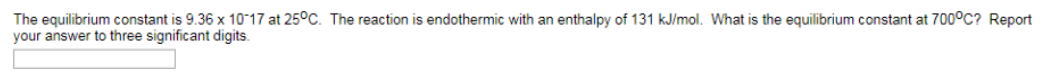Chemistry Practice Problems Equilibrium Expressions Practice Problems Solution: The equilibrium constant is 9.36 x 10-17 at 25 °C....

# Solution: The equilibrium constant is 9.36 x 10-17 at 25 °C. The reaction is endothermic with an enthalpy of 131 kJ/mol. What is the equilibrium constant at 700 °C? Report to three significant digits.

###### Problem

The equilibrium constant is 9.36 x 10-17 at 25 °C. The reaction is endothermic with an enthalpy of 131 kJ/mol. What is the equilibrium constant at 700 °C? Report to three significant digits.View Complete Written Solution

Equilibrium Expressions

Equilibrium Expressions

#### Q. What is the numerator for the equilibrium expression, Kc, for the following chemical equilibrium? Cu2+ (aq) + 4NH3 (aq) = Cu(NH3)42+ (aq) a. [Cu(NH3)4...

Solved • Wed Aug 01 2018 13:07:19 GMT-0400 (EDT)

Equilibrium Expressions

#### Q. Nitric oxide and bromine were allowed to react in a sealed container. When equilibrium was reached PNO = 0.526 atm = 1.59 atm, and PNOBr = 12.2 atm. C...

Solved • Wed Aug 01 2018 12:59:55 GMT-0400 (EDT)

Equilibrium Expressions

#### Q. At a certain temperature, the equilibrium constant for the following chemical reaction is 0.00145. At equilibrium, the concentration of AB is 2.725 M,...

Solved • Tue Jul 31 2018 13:37:29 GMT-0400 (EDT)

Equilibrium Expressions

#### Q. The equilibrium between hemoglobin and oxyhemoglobin in the blood can be represented by the following reaction. Write the form of the equilibrium cons...

Solved • Mon Jul 23 2018 15:56:07 GMT-0400 (EDT)